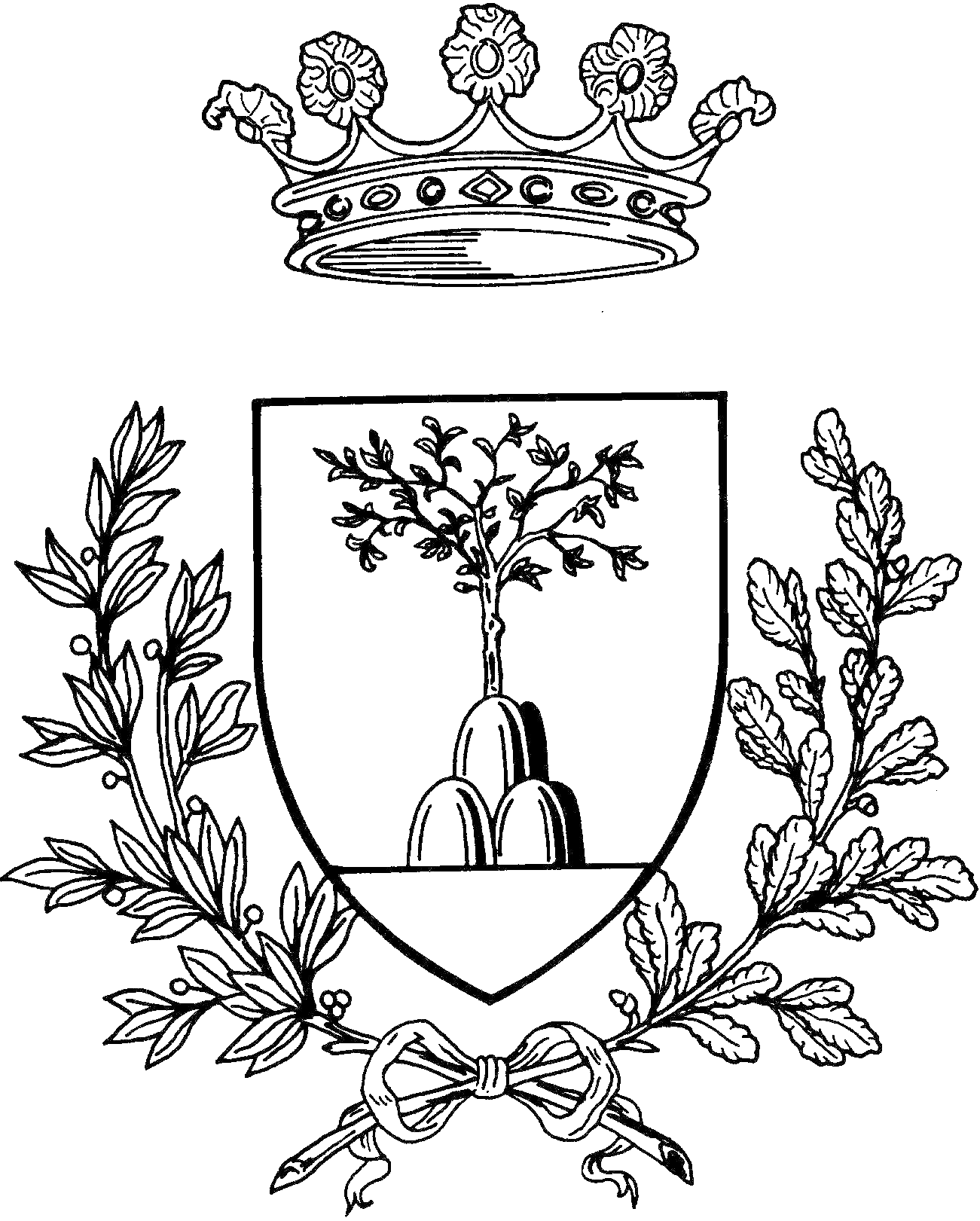- The Riemann-Hurwitz theorem- Lecture notes: "Toric varieties, Mori dream spaces and Cox rings " (IMPA-UFF, 2020)- Lecture notes: "Topics in algebraic geometry: Cox rings" (IMPA, 2015)- Mori Dream Spaces, log Fano varieties and moduli spaces of rational curves- On the automorphisms groups of moduli spaces of curves (French)- The 2-Secant varieties of the Veronese embedding- Exercises of Algebraic Geometry I- Some solved exercises from Hartshorne's book- Local Systems, Representations of the Fundamental Group and Flat Connections - Colimits and Shaves (Italian)- Elliptic Curves (Italian)- Nakayama's Lemma- Riemann Surfaces- Quantum Algebras- Cubic Surfaces (Italian)- Riemann Map Theorem (Italian)- Some computations in Algebraic Geometry with MacAulay2 and Bertini- The Kodaira Embedding Theorem- Polynomials Decomposition as Sums of Powers- Moduli of Curves- A note on the Yoneda Lemma - Grothendieck Riemann Roch Theorem- Moduli of Stable Maps, Gromov-Witten Invariants and Quantum Cohomology